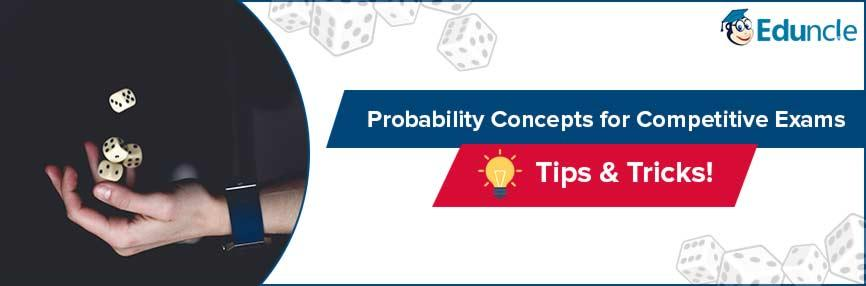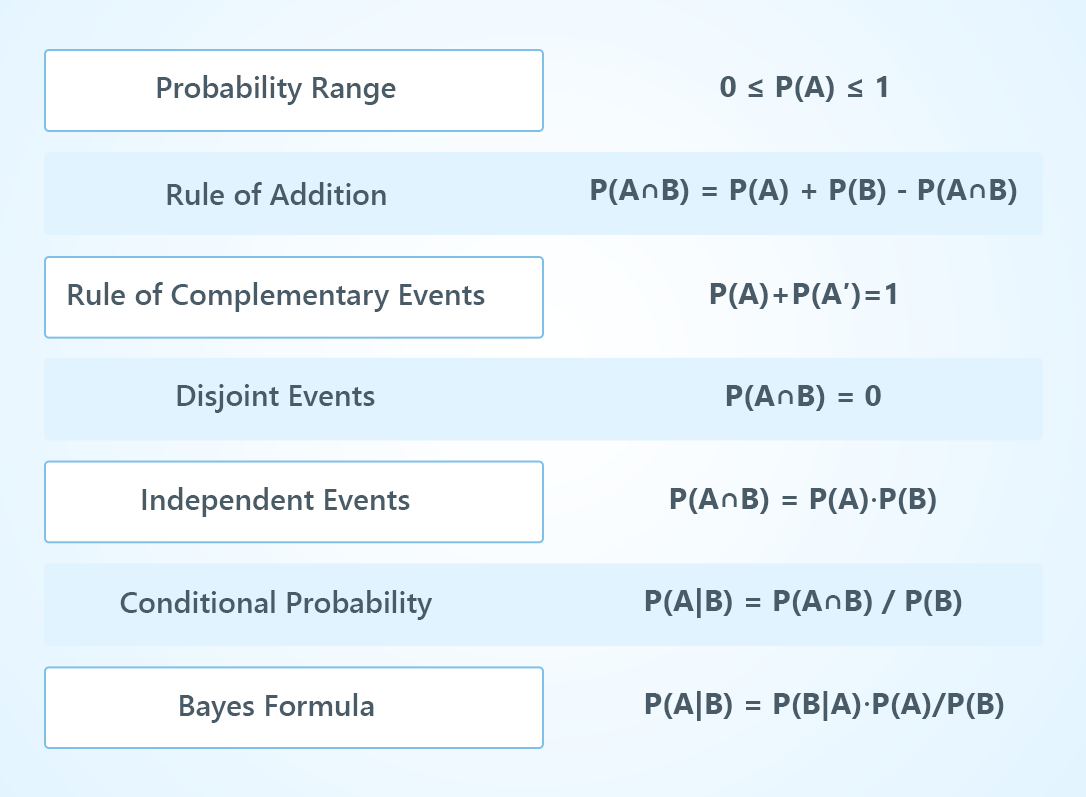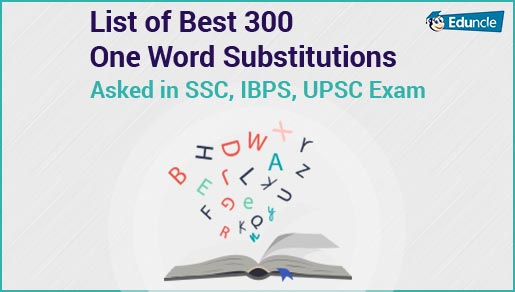Government Jobs FollowRavi @Eduncle posted an Article
May 24, 2019 • 2:53 pm

# How to Solve Probability Questions of Competitive Exams? Tips & Tricks‘Probability’ the most common topic in almost all the government jobs exams like Banking, Civil Services, Public Service Commission, Government Lecturer, Defence Services, Railways, etc.

Just like other topics of Aptitude section, Probability is also considered to be important topic to score well in exam.

Candidates who wish to score well in this topic should read this blog to know about the common factors like Probability Distribution, Formulas, Statistics etc.

Below in this blog, you will get to know about all the terminologies that are used to solve Probability problems.

Scroll down to acquire complete knowledge.

Targeting Upcoming SSC Exams 2018-2019? Get Free Study Materials and Previous Year Question Papers. Download Now.

## What is Probability? Know the Complete Probability Definition Here

The Probability definition might be confusing, but when you understand it you can easily solve even the toughest problems.

We can define the word ‘Probability’ as - A measure of how likely an event is to occur’.

Probability is the number of ways of achieving success to the total number of possible outcomes.

Conclusively we can say that ‘Probability is the measure of Uncertainty’.

To clear the concept of solving Probability Questions, there are also some basic terminologies which need to be considered.

Below you can check all the relative information to dive deep in Probability concepts.

## Basic Terminologies Used in Probability

Understanding the basic terminologies of Probability is important to start attempting the questions.

Below you we have described importance terms that are considered while attempting a Probability Question.

#### Experiment –

It’s an event or we can say it’s an operation which provides some well-defined outcomes.

#### Random Experiment –

It’s a type of experiment, in which all the possible outcomes are known, but we can not predict the exact output in advance.

The most common examples of Random experiment are –

Rolling an unbiased dice

Drawing a card from well-shuffled cards

Coin flipping

Picking up a colored ball from a bag containing the differently colored ball.

#### Event –

Outcomes that we get after performing an experiment are known as events. There are different types of events which are elaborated below.

Compound Event – Combination of two or more elementary events is called a compound event.

Elementary Event – All the possible outcomes after repeating a random experiment under exact conditions is known as Elementary Event.

Mutually Exclusive Events – Flipping a coin means either head will come, or tail will come. Both these cases are counted in Mutually Exclusive Events.

Favorable Events – Total desired outcomes of an elementary event are counted in Favourable Event.

Independent Events – If the outcome of one event is not affecting the outcome of other events then both the events are called independent events.

Don’t be in a hurry to solve Probability questions, just read the above-mentioned terms and clear the basics of Probability.

Now let’s have a look at all the important Probability Formulas which are used to solve the questions in all government jobs exams.

Start Preparation of Bank Exams 2019 with Free Study Stuff - Download Now!

## Important Probability Formulas List

Here we have listed the important Probability formulas that can help you to solve the problems easily.

Scroll down to check the list.Start Preparation of Bank Exams 2018-19 with Free Study Stuff & Video Lectures - Download Now!

## Most Probably Cases Discussed in Probability – Dice, Coins, & Cards!

Candidates who are preparing for the competitive exams like IBPS PO, IBPS Clerk, SSC CGL, SSC CHSL, Railway and for any other exam, should know that which kind of Probability questions can be asked.

Here we are sharing the most common type of Probability cases for the competitive exams.

Enhance your knowledge in Probability here –

### 1. Probability Problems on Dice

This type of Probability Questions is asked on the basis of rolling dice with six sided dots – 1,2,3,4,5 and 6.

When one dice is rolled, the number of possibilities is 6.

When the two dice are rolled together, the number of possibilities is 6*6 = 36.

To check the outcomes of two dice, below we have shared the sample space of Probability.

 1 2 3 4 6 6 1 (1,1) (1,2) (1,3) (1,4) (1,5) (1,6) 2 (2,1) (2,2) (2,3) (2,4) (2,5) (2,6) 3 (3,1) (3,2) (3,3) (3,4) (3,5) (3,6) 4 (4,1) (4,2) (4,3) (4,4) (4,5) (4,6) 5 (5,1) (5,2) (5,3) (5,4) (5,5) (5,6) 6 (6,1) (6,2) (6,3) (6,4) (6,5) (6,6)

Here in the table, the outcomes (1,1), (2,2), (3,3), (4,4), (5,5) and (6,6) are known as doublets and the pair (1,2) and (2,1) are different outcomes.

### 2. Probability Problems on Coins

When we throw a coin in the air there are two conditions that can occur. Whether it lands with head or with a tail.

Most of the time questions are asked on Coins problem.

Probability questions on coins can be asked in three different types –

#### When only One Coin Flipped –

In this case, total events will be 2 and Probability P(E) is = 1/2.

#### When Two Coins are Flipped –

The sample space will be – {(H,T), (T,H), (H,H), (T,T)}

If the question is asked “What is the probability that both the coins shows head?”, The possibility of two heads will be decided as P(H,H) = n(E)/n(S)

Putting the values in formula, we can say that the probability when both the coins show head is 1/4.

#### When Three Coins are Flipped –

This time the sample space will be changed as - {(H,H,H), ( T,T,T), (H,H,T), ( H,T,H), (T,H,H), (H,T,T), (T,H,T) ,(T ,T, H)}

Here, we see the probability that both the coins show tails will be P(E) is = 3/8.

### 3.Probability Problems on Cards

Questions are also asked on the basis of a well-shuffled deck of 52 Playing Cards Probability.

Here you can clear all the concepts to attempt the Playing Cards Probability Questions.

The deck of 52 playing cards is divided into 4 suits of 13 cards each i.e. –

Hearts (Red Cards)

Diamonds (Red Cards)

Clubs (Black Cards)

Each suit of cards contains an ace, king, queen, jack, 10,9,8,7,6,5,4,3, and 2.

There are total 12 face cards (King, Queen and Jack) in a deck of playing cards.

Let’s clear it with a simple example –

Question – Find the probability of a jack drawn from a deck of 52 cards?

Solution

The formula is same to get the Probability i.e.

Number of Favorable Outcomes/Total Number of Possible Outcome

Here we can see that the number of favorable outcomes of ‘a jack’ is 4 out of 52 cards and the total number of possible outcomes is 52.

So, the Probability of drawing a jack is = 4/52.

Prepare for Upcoming SSC Exams! Download Here to get free study materials PDFs and Sample Papers for all exams.

Working on small topics will lead you with a great score in any competitive exams.

We hope that the blog helped you to clear all your doubts like What is Probability and which type of Probability questions asked in the exams.

Still, if you have any query regarding Probability or with any other topics, feel free to ask.

You Might Also Like